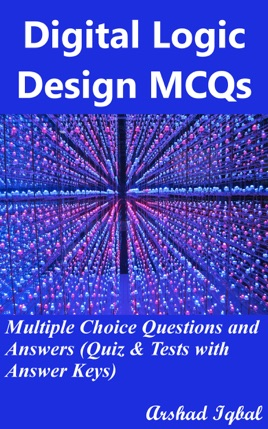• \$4.99

## Publisher Description

"Digital Logic Design MCQs: Multiple Choice Questions and Answers (Quiz & Tests with Answer Keys)" provides mock tests for competitive exams to solve 700 MCQs. "Digital Logic Design MCQ" pdf to download helps with theoretical, conceptual, and analytical study for self-assessment, career tests. Digital logic design quizzes, a quick study guide can help to learn and practice questions for placement test preparation.
"Digital Logic Design Multiple Choice Questions and Answers (MCQs)" pdf to download is a revision guide with a collection of trivia quiz questions and answers pdf on topics: Algorithmic state machine, asynchronous sequential logic, binary systems, Boolean algebra and logic gates, combinational logics, digital integrated circuits, DLD experiments, MSI and PLD components, registers counters and memory units, simplification of Boolean functions, standard graphic symbols, synchronous sequential logics to enhance teaching and learning.
Digital Logic Design Quiz Questions and Answers pdf also covers the syllabus of many competitive papers for admission exams of different universities from computer science textbooks on chapters:

Algorithmic State Machine Multiple Choice Questions: 50 MCQs. Asynchronous Sequential Logic Multiple Choice Questions: 50 MCQs. Binary Systems Multiple Choice Questions: 50 MCQs. Boolean Algebra and Logic Gates Multiple Choice Questions: 50 MCQs. Combinational Logics Multiple Choice Questions: 50 MCQs. Digital Integrated Circuits Multiple Choice Questions: 50 MCQs. DLD Experiments Multiple Choice Questions: 150 MCQs. MSI and PLD Components Multiple Choice Questions: 50 MCQs. Registers Counters and Memory Units Multiple Choice Questions: 50 MCQs. Simplification of Boolean Functions Multiple Choice Questions: 50 MCQs. Standard Graphic Symbols Multiple Choice Questions: 50 MCQs. Synchronous Sequential Logics Multiple Choice Questions: 50 MCQs.

"Asynchronous Sequential Logic MCQ" pdf chapter covers quiz about introduction to asynchronous sequential logic, analysis of asynchronous sequential logic, circuits with latches, design procedure of asynchronous sequential logic, and transition table.
"Binary Systems MCQ" pdf chapter covers quiz about binary systems problems, complements in binary systems, character alphanumeric codes, arithmetic addition, binary codes, binary numbers, binary storage and registers, code, decimal codes, definition of binary logic, digital systems, error detection code, gray code, logic gates, number base conversion, octal and hexadecimal numbers, radix complement, register transfer, signed binary number, switching circuits, and binary signals.
"Boolean Algebra and Logic Gates MCQ" pdf chapter covers quiz about basic definition of Boolean algebra, digital logic gates, axiomatic definition of Boolean algebra, basic algebric manipulation, theorems and properties of Boolean algebra, Boolean functions, complement of a function, canonical and standard forms, conversion between canonical forms, standard forms, integrated circuits, logical operations, operator precedence, product of maxterms, sum of minterms, and Venn diagrams.
"Combinational Logics MCQ" pdf chapter covers quiz about introduction to combinational logics, full adders, design procedure in combinational logics, combinational logics analysis procedure, adders, Boolean functions implementations, code conversion, exclusive or functions, full subtractors, half adders, half subtractors, multi-level NAND circuits, multi-level nor circuits, subtractors in combinational logics, transformation to and-or diagram, and universal gates in combinational logics.
"Synchronous Sequential Logics MCQ" pdf chapter covers quiz about introduction to synchronous sequential logic, flip-flops in synchronous sequential logic, clocked sequential circuits, clocked sequential circuits analysis, design of counters, design procedure in sequential logic, flip-flops excitation tables, state reduction and assignment, and triggering of flip-flops.